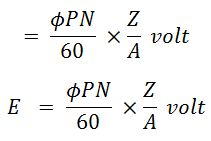# E.M.F Equation of DC Generator

We know that the working principle of dc generator, that when conductors begin to cut the magnetic lines of force and therefore, the e.m.f. induces in the conductors according to 'Faraday's Law of Electromagnetic Induction'.

The value of induced e.m.f. depends upon the lengths of the conductor, the magnetic field strength, and the speed at which the coil rotates. Let us see the equation for induced e.m.f.

#### Let,φ = Flux per pole in Weber.Z = Total number of armature conductors.N = Armature rotation in revolution per minute (rpm).P = Number of poles.A = Number of parallel paths in armature.E = EMF induced in any parallel path or generated emf.

According to Faraday's law of Electromagnetic induction. Average emf generated per conductors,

#### Time taken for one revolution,dt = 60/N secE.m.f generated per conductor,Therefore, the total emf E generated between the terminals if given as,E = Average e.m.f generated per conductor × Number of conductor in each parallel pathWhere,A = P for lap winding.A = 2 for wave winding.

Do not enter any spam links and messages